# Field of view and focal length

Written by Paul Bourke
April 2003

Camera and photography people tend to talk about lens characteristics in terms of "focal distance" while those involved in synthetic image generator (such as raytracing) tend to think in terms of field of view for a pinhole camera model. The following discusses (an idealised at least) way to estimate the field of from the focal distance. view

The focal length of a lens is an inherent property of the lens, it is the distance from the center of the lens to the point at which objects at infinity focus. Note: this is referred to as a rectilinear lens.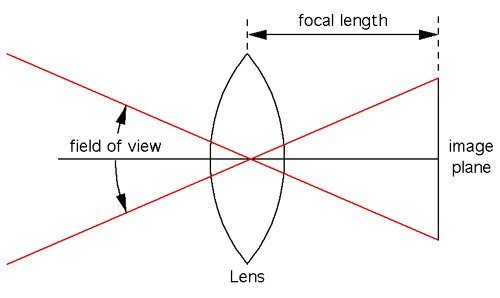That there are three possible ways to measure field of view: horizontally, vertically, or diagonally. The horizontal field of view will be used here, the other two can be derived from this. From the figure above, simple geometry gives the horizontal field of view

horizontal field of view = 2 atan(0.5 width / focallength)

where "width" is the horizontal width of the sensor (projection plane). So for example, for a 35mm film (frame is 24mm x 36mm), and a 20mm (focal length) lens, the horizontal FOV would be almost 84 degrees (vertical FOV of 62 degrees). The above formula can similarly be used to calculate the vertical FOV using the vertical height of the film area, namely:

vertical field of view = 2 atan(0.5 height / focallength)

So for example, for 120mm medium format film (height 56mm) and the same 20mm focal length lens as above, the vertical field of view is about 109 degrees.

# Changing to/from vertical/horizontal field of view

Written by Paul Bourke
March 2000

PovRay measures its field of view (FOV) in the horizontal direction, that is, a camera FOV of 60 is the horizontal field of view. Some other packages (for example OpenGL gluPerspective()) measure their FOV vertically. When converting camera settings from these other applications one needs to compute the corresponding horizontal FOV if one wants the views to match.

It isn't difficult, here's the solution. By calculating the distance from the camera to the center of the screen one gets the following:

height / tan(vfov/2) = width / tan(hfov/2)

Solving this gives

hfov = 2 atan[ width tan(vfov/2) / height]

Or going the other way
vfov = 2 atan[ height tan(hfov/2) / width]Where width and height are the dimensions of the screen. For example, a camera specification to match an OpenGL camera FOV of 60 degrees might be:

```camera {
location <200,3600,4000>
up y
right -width*x/height
angle 60*1.25293
sky <0,1,0>
look_at <200+10000*cos(-clock),3600+2500,4000+10000*sin(-clock)>
}
```

# Lens Correction and Distortion

Written by Paul Bourke
April 2002

The following describes how to transform a standard lens distorted image into what one would get with a perfect perspective projection (pin-hole camera). Alternatively it can be used to turn a perspective projection into what one would get with a lens.

To illustrate the type of distortion involved consider a reference grid, with a 35mm lens it would look something line the image on the left, a traditional perspective projection would look like the image on the right.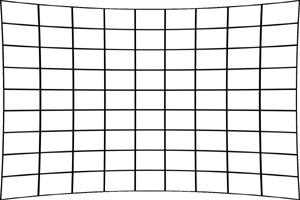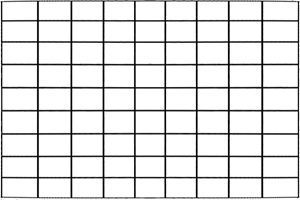The equation that corrects (approximately) for the curvature of an idealised lens is below. For many lens projections ax and ay will be the same, or at least related by the image width to height ratio (also taking the pixel width to height relationship into account if they aren't square). The more lens curvature the greater the constants ax and ay will be, typical value are between 0 (no correction) and 0.1 (wide angle lens). The "||" notation indicates the modulus of a vector, compared to "|" which is absolute value of a scalar. The vector quantities are shown in red, this is more important for the reverse equation.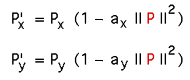Note that this is a radial distortion correction. The matching reverse transform that turns a perspective image into one with lens curvature is, to a first approximation, as follows.In practice if one is correcting a lens distorted image then one actually wants to use the reverse transform. This is because one doesn't normally transform the source pixels to the destination image but rather one wants to find the corresponding pixel in the source image for each pixel in the destination image.

Note that in the above expression it is assumed one converts the image to a normalised (-1 to 1) coordinate system in both axes.

 For example: Px = (2 i - width) / width Py = (2 j - height) / height and back the other way i = (Px + 1) width / 2 j = (Py + 1) height / 2Example 1 Original photo of reference grid with 35mm camera lens is shown on the right. The corrected image is given below and the distortion reapplied is at the bottom right. Note the transformation is a contraction (for positive ax and ay), the grey region corresponds to points that map from outside the original image. Original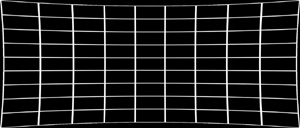Forward transformReverse applied to forward transform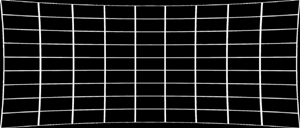Example 2 Original photo of reference grid with 50mm camera lens is shown on the right align with the corrected version below and the redistorted version bottom right. Original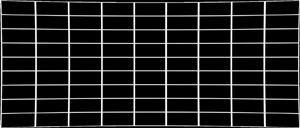Forward transform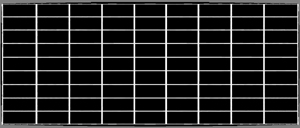Reverse applied to forward transform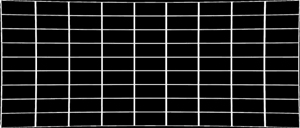Example code

"Proof of concept code" is given here: map.c As with all image processing/transformation processes one must perform antialiasing. A simple supersampling scheme is used in the above code, a better more efficient approach would be to include bicubic interpolation.

The effect of adding lens distortion to the image is shown below for a perspective projection of a Menger sponge by Angelo Pesce. The image on the left is the original from PovRay, the image on the right is the lens affected version. (distort.c)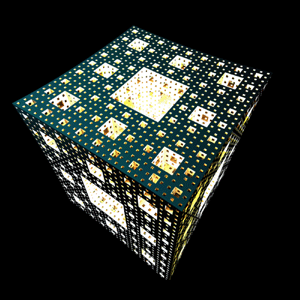References

F. Devernay and O. Faugeras. SPIE Conference on investigative and trial image processing. SanDiego, CA, 1995. Automatic calibration and removal of distortion from scenes of structured environments.

H. Farid and A.C. Popescu. Journal of the Optical Society of America, 2001. Blind removal of Lens Distortion

R. Swaminatha and S.K. Nayer. IEEE Conference on computer Vision and pattern recognition, pp 413, 1999. Non-metric calibration of wide angle lenses and poly-cameras

G. Taubin. Lecture notes EE-148, 3D Photography, Caltech, 2001. Camera model for triangulation

# Non-linear Lens Distortion

With an example using OpenGL (lens.c, lens.h)

Written by Paul Bourke
August 2000

The following illustrates a method of forming arbitrary non linear lens distortions. It is straightforward to apply this technique to any image or 3D rendering, examples will be given here for a few mathematical distortion functions but the approach can use any function, the effects are limited only by your imagination. At the end an OpenGL application is given that implements the technique in real-time (given suitable OpenGL hardware and texture memory).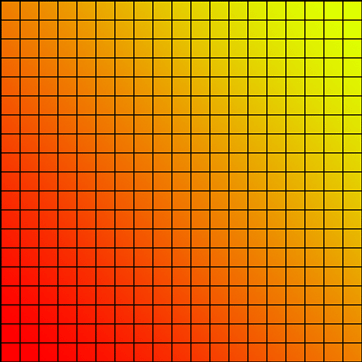This is the sample input image that will be used to illustrate a couple of different distortion functions. Consider the linear function below: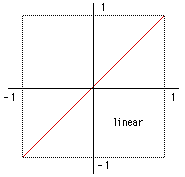The horizontal axes is the coordinate in the new image, the vertical axis is the coordinate in the original image. To find the corresponding pixel in the new image one locates the value on the horizontal axis and moves up to the red line and reads off the value on the vertical axis. The linear function above would result in an output image that looks the same as the input image. sine A more interesting example is based upon a sine curve. You should be be able to convince yourself that this function will stretch values near +1 and -1 while compressing values near the origin. An important requirement for these distortion functions is they need to be strictly one-to-one, that is, there is a unique vertical value for each horizontal value (and visa-versa). If image flipping is disallowed then this implies the distortion function is always increasing as one moves from left to right along the horizontal axis.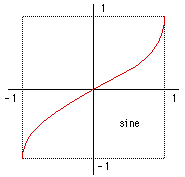There are two ways of applying this function to an image, the first shown on the left in each example below applies the function to the horizontal and vertical coordinates of the image. The example on the right applies the function to the radius from the center of the image, the angle is undistorted.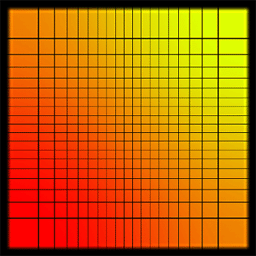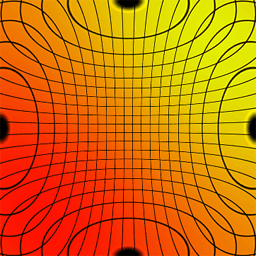square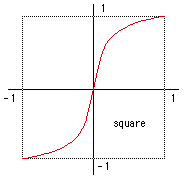There are a number of ways the image coordinates are mapped onto the function range. The approach used here was to scale and translate the image coordinates so that 0 is in the center of the image and the bounds of the image range from -1 to +1. This is done twice, one to map the output image coordinates to the -1 to +1 range, the function is then applied, and then the inverse transformation maps the -1 to +1 range onto the range in the input image. So if iout and jout are the coordinates of the output image, and wout and hout the output image dimensions, then the mapping onto the -1 to +1 range is xout = iout / (wout/2) - 1, and yout = jout / (hout/2) - 1 Applying the function to xin and yin gives xnew and ynew. The inverse mapping from the xnew and ynew gives iin and jin (the index in the input image with a width of win and hin) is just iin = (xnew + 1) * (win/2), and jin = (ynew + 1) * (hin/2) Given iin and jin the colour in the input image can be applied to pixel iout, jout in the output image.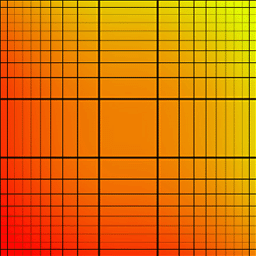asin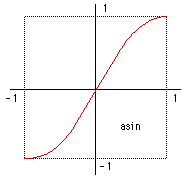Applying the function to polar coordinates is only slightly different. The radius and angle of a pixel is computed based up xout and yout. The radius lies between 0 and 1 so the positive half of the function is used to transform it. The pixel coordinates in the input image are calculated using the new radius and the unchanged angle. Using the conventions above: rout = sqrt(xout2 + yout2), and angleout = atan2(yout,xout) The transformation is applied to rout to give rnew, xnew and ynew is calculated as xin = rnew cos(angleout), and yin = rnew sin(angleout) iin and jin are calculated as before from xin and yin. Note that in both cases (distorting the Cartesian coordinates or polar coordinates) it is possible for there to be an unmappable region, that is, coordinates in the new image which when distorted lie outside the bounds of the input image.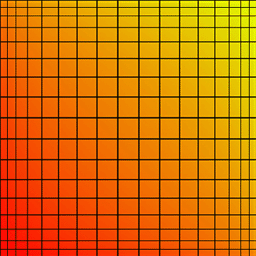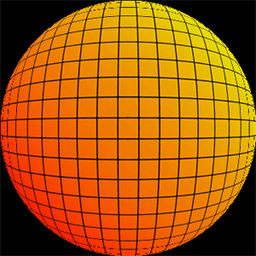Notes on resolution

Some parts of the image are compressed and other parts inflated, the inflated regions need a higher input image resolution in order to be represented without aliasing effects. The above transformations cope with the input and output images being different sizes, normally the input image needs to be much larger than the output image. To minimise aliasing the input image should be larger by a factor equal to the maximum slope of the distorting function. There are no noticeable artefacts in these example because the input image was 10 times larger than the output image.

OpenGL

This OpenGL example implements the distortion functions above and distorts a grid and a model of a pulsar. It can readily be modified to distort any geometry. The guts of the algorithm can be found in the HandleDisplay() function. It renders the geometry as normal, then copies the resulting image and uses it as a texture that is applied to a regular grid. The texture coordinates of this grid are formed to give the appropriate distortion. (lens.c, lens.h) The left button rotates the camera around the model, the middle button rolls the camera, the right button brings up a few menus for changing the model and the distortion type. It should be quite easy for you to add your own geometry and to experiment with other distortion functions.
This example expects the Glut library to be available.

Improvements and exercises for the reader
• An improvement would be to render the texture at a larger size so that there is more resolution at those parts of the distorted image that are inflated. The note above on image resolution is clearly observed in this OpenGL implementation.

• Some OpenGL implementations will support non square power of 2 textures in which case the restrictions on the window size can be removed. Many implementations also support non square power of 2 textures if mipmapping is enabled.

• If you'd like to try some other interesting distortion functions then experiment with the following.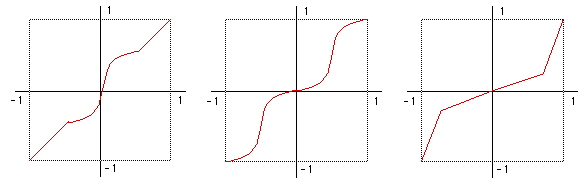The first is similar to the fisheye lens people used to attach to the window of their ute. The second is similar to the wave-like distorting mirrors found at carnival shows.

Feedback from Daniel Vogel

One thing you might want to consider is using glCopyTexSubImage2D instead of doing a slow glReadPixels. Using the first allows me to play UT smoothly with distortion enabled. glReadPixels is a very slow operation on consumer level boards. And until there is a "rendering to texture" extension for OpenGL taking the texture directly from the back buffer is the fastest way - and it even is optimized.

# Computer Generated Camera Projections and Lens Distortion

Written by Paul Bourke
September 1992

Most users of 3D modelling and rendering software are familiar with parallel and perspective projections when they generate wire frame, hiddenline, simple shaded or highly realistic rendered images. It is possible to mathematically describe many other projections some of which may not be available, feasible, or even possible with conventional photographic equipment. Some of these techniques will be illustrated and discussed here using as an example a computer based model of Adolf Loos' Karntner bar. The 3D model was created by Matiu Carr in 1992 at the University of Auckland's School of Architecture, using Radiance.

 This image is an example of a conventional perspective projection (90 degree FOV, 17mm) of the sort offered by most rendering packages. The user is able to specify the position and direction of a virtual camera in the scene as well as other camera attributes such as FOV and depth of field.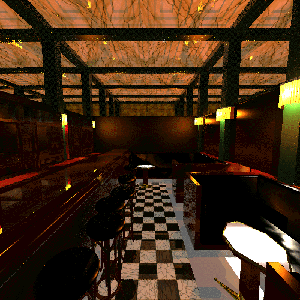Figure: Perspective 90

 Virtual cameras don't suffer from some of the restrictions imposed by a real camera. This is an image using a 140 degree FOV which corresponds to approximately a 6mm lens.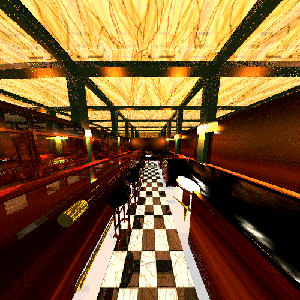Figure: Perspective 140

 A hemispherical fisheye (180 degrees) maps the front hemisphere of the projection sphere onto a planar circular area on the image plane. The image shows everything in front of the camera position.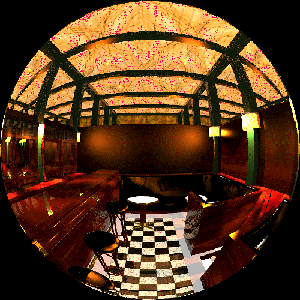Figure: Hemisphere 180

 This 360 degree fisheye is an unwrapping of the scene projected onto a sphere onto a circular image on the projection plane. Those parts of the scene behind the camera are severely distorted, so much so that the circumference of the image maps to a single point behind the camera.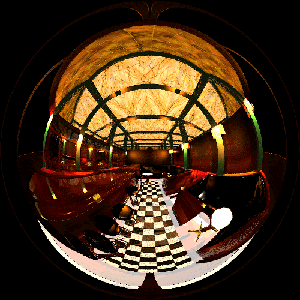Figure: Fisheye 360

The following is a 180 degree (vertically) by 360 degree (horizontally) angular fisheye. It unwraps a strip around the projection sphere onto a rectangular area on the image plane. The distance from the centre of the image is proportional to the angle from the viewing direction vector.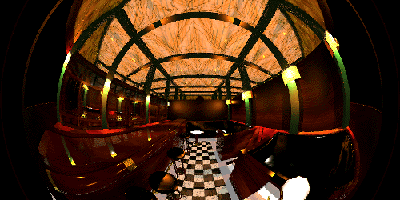Figure: Fisheye 180

90 degree (vertically) by 180 degree (horizontally) angular fisheye.Figure: Fisheye 90

A panoramic view is another method of creating a 360 degree view, it removes vertical bending but introduces other forms of distortion. This is created by using a virtual camera that has a 90 degree vertical field of view and a 2 degree horizontal field of view. The virtual camera is rotated about the vertical axis in 2 degree steps, the resulting 180 image strips are pasted together to form the following image.Figure: Panoramic 360

Some other "real" examples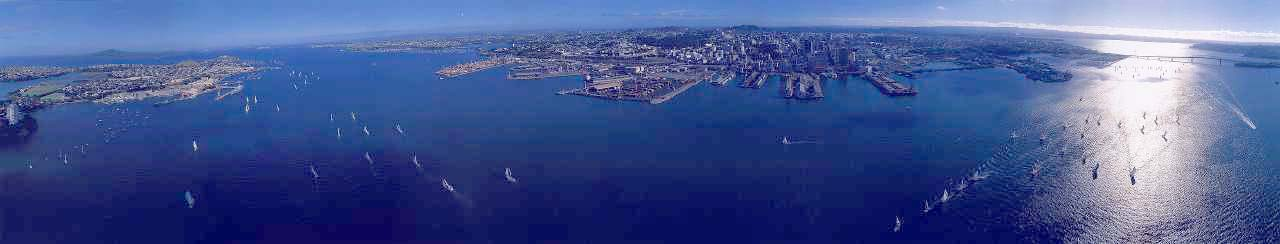180 degree panoramic view of Auckland Harbour.360 by 180 degree panoramic view created by a camera developed at Monash University, Melbourne.